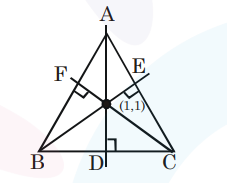# Let the equations of two sides of a triangle be

Question:

Let the equations of two sides of a triangle be $3 x$ $-2 y+6=0$ and $4 x+5 y-20=0$. If the orthocentre of this triangle is at $(1,1)$, then the equation of its third side is :

1. $122 y-26 x-1675=0$

2. $26 x+61 y+1675=0$

3. $122 y+26 x+1675=0$

4. $26 x-122 y-1675=0$

Correct Option: , 4

Solution:Equation of $\mathrm{AB}$ is

$3 x-2 y+6=0$

equation of $\mathrm{AC}$ is

$4 x+5 y-20=0$

Equation of BE is

$2 x+3 y-5=0$

Equation of CF is $5 x-4 y-1=0$

$\Rightarrow$ Equation of $\mathrm{BC}$ is $26 \mathrm{x}-122 \mathrm{y}=1675$